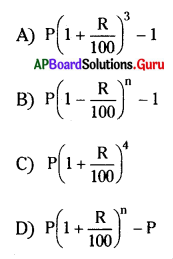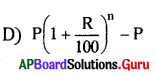Practice the AP 8th Class Maths Bits with Answers Chapter 5 Comparing Quantities Using Proportion on a regular basis so that you can attempt exams with utmost confidence.

## AP State Syllabus 8th Class Maths Bits 5th Lesson Comparing Quantities Using Proportion with Answers

Question 1.
Which of the following is a golden ratio?
A) 25 : 1
B) 1.615 : 1
C) 1.5 : 1
D) 1 : 2
B) 1.615 : 1

Question 2.
The compound ratio of a : b and c : d is
A) ab : cd
B) be : bd
C) ac : bd
C) ac : bdQuestion 3.
The compound ratio of 5 : 7 and inverse 2 : 9 is
A) 45 : 14
B) 10 : 63
C) 63 : 10
D) 14 : 45
A) 45 : 14

Question 4.
30% of 40 =
A) 22
B) 7
C) 12
D) 48
C) 12

Question 5.
Increase in percent of 2 times of a number is
A) 200%
B) 100%
C) 300%
D) 50%
B) 100%

Question 6.
The cost of one pair of shoes is ₹550, if 10% discount is allowed on, then the SP. of shoes is
A) ₹505
B) ₹495
C) ₹485
D) ₹475
B) ₹495

Question 7.
The marked price of a cycle is ₹3600 and it S.P. is ₹3312 then discount% is
A) 8%
B) 9%
C) 6%
D) 7%
A) 8%

Question 8.
The cost of one pair of clothes is ₹450. If 6% of sales tax is allowed on it then the bil has to pay
A) ₹ 57
B) ₹ 477
C) ₹ 46
D) ₹ 423
B) ₹ 477

Question 9.
In A = P (1 + )n , ‘R’ stands for
A) Principal
B) Time
C) C.I.
D) Rate of interest
D) Rate of interestQuestion 10.
Selling Price =
A) M.P – discount
B) Discount – M.P
C) M.P – Profit
D) None
A) M.P – discount

Question 11.
The M.P. of an item is ₹ 1600 and allowed adiscountof6%to the purchaser then SP is ……………
A) ₹ 1107
B) ₹ 1105
C) ₹ 504
D) ₹ 1504
D) ₹ 1504

Question 12.
Profit Percentage = …………………..
A)  × 100
B)  × 100
C) 
D) None
B)  × 100

Question 13.
If two cycles are sold on equal price. He get 10% profit on the first and 10% loss on the second then his overall profit or loss percent = ………………..
A) Loss percentage 1%
B) Profit percentage 2%
C) Loss percentage 3%
D) None
A) Loss percentage 1%

Question 14.
Simple Interest, I = …………………
A) $$\frac{100}{\mathrm{PTR}}$$
B) $$\frac{\mathrm{PT}^{2} \mathrm{R}}{100}$$
C) $$\frac{\mathrm{PTR}}{100}$$
D) $$\frac{P}{100 \mathrm{~T}}$$
C) $$\frac{\mathrm{PTR}}{100}$$

Question 15.
S.I on ₹ 500 at the rate of 5% of 2 years is ………………
A) ₹ 16
B) ₹ 50
C) ₹ 20
D) ₹ 90
B) ₹ 50

Question 16.
Compound interest = ………………..Question 17.
The number of time periods for compounded every 6 months, for 2$$\frac {1}{2}$$ years is …………………
A) 7
B) 6
C) 5
D) 10
C) 5Question 18.
The C.I on ₹ 5000 at the rate of 8% for 2 years is ……………….
A) ₹ 832
B) ₹ 238
C) ₹ 161
D) ₹ 169
A) ₹ 832

Question 19.
The population of Krosur village in 2012 is 6250. If it increases at rate of 8% then the population of the village in 2014 is ………………
A) 7920
B) 7190
C) 7490
D) 7290
D) 7290

Question 20.
P is the principle, R% is rate and time period is T, Simple Interest S and Compound Interest is C then observe the following cases.
(i) C > S
(ii) C = S
(iii) C < S
A) Only (i) is true
B) Either (i) or (ii) is true
C) Either (ii) or (iii) is true
D) Only (ii) is true
A) Only (i) is true

Question 21.
The MP of an item is ₹ 80 and it sold is for X 76 then the discount percentage is …………………….
A) 6%
B) 5%
C) 15%
D) 20%
B) 5%

Question 22.
Navya bought a scooter for ₹ 36,450 and paid a tax of 9% then how much amount she paid in total ?
A) ₹ 19,370
B) ₹ 39,170.5
C) ₹ 39,0470
D) ₹ 39,730.5
D) ₹ 39,730.5

Question 23.
After allowing a discount of 20% the cost of a shirt is ₹ 1,120 then the MP is ………………
A) ₹ 1440
B) ₹ 1410
C) ₹ 2310
D) ₹ 1443
A) ₹ 1440

Question 24.
The cost of a T.V with VAT 5% is ₹ 26,250 then the cost of that T.V is
A) ₹ 21,000
B) ₹ 52,000
C) ₹ 25,000
D) ₹ 16,000
C) ₹ 25,000Question 25.
40% of (100 – 20% of 300) = …………………..
A) 19
B) 10
C) 26
D) 16
D) 16

Question 26.
The new salary of a person after increasing 10% is ₹ 1,54,000 then the old salary was ………………..
A) ₹ 1,70,001
B) ₹ 1,90,000
C) ₹ 1,30,000
D) ₹ 1,40,000
D) ₹ 1,40,000

Question 27.
On a Monday 845 people visited a park and on Tuesday 169 people visited then how much decrease percentage is observed in visitors ?
A) 20%
B) 30%
C) 70%
D) 80%
D) 80%

Question 28.
3 : 4 = …………….. %
A) 70
B) 75
C) 65
D) 80
B) 75

Question 29.
66 $$\frac {2}{3}$$ % = …………………
A) 2 : 3
B) 3 : 2
C) 1 : 4
D) 41
A) 2 : 3

Question 30.
72% of 25 students got good narks in Mathematics, then how maiy people did not score good marks ?
A) 13
B) 11
C)10
D) 7
D) 7

Question 31.
After spending 75% in his Dtal money Ravi was left with Rs 600/. Then how much money he had originally ?
A) ₹ 800
B) ₹ 1600
C) ₹ 2400
D) ₹ 120
C) ₹ 2400Question 32.
P = ₹ 10,000, R = 4%, n = then A = ₹ ……………….
A) 10,816
B) 11,86
C) 10,861
D) 12,81
A) 10,816

Question 33.
P = ₹ 12,000, R = 6% = 2 years then I = ?
A) ₹ 1320
B) ₹ 1,120
C) ₹ 1,220
D) ₹ 1,440
D) ₹ 1,440

Question 34.
The cost of a scooter is ₹ 42,000 and the depreciation value for every year is 8% then the value of scooter after 1 year is ……………….
A) ₹ 18,600
B) ₹ 38,640
C) ₹ 39,469
D) ₹ 18,460
B) ₹ 38,640

Question 35.
Raju took some amount from a bank at 10$$\frac {1}{2}$$% per 1 year. He paid ₹ 1863.75 as interest after 2$$\frac {1}{2}$$ years. Then how much amount was initially taken ?
A) ₹ 6,500
B) ₹ 1,600
C) ₹ 6,800
D) ₹ 7,100
D) ₹ 7,100

Question 36.
On certain amount simple interest is $$\frac {16}{25}$$ th part of the amount taken then the time and rate of interest is ……………….. (Numerically same)
A) 8
B) 9
C) 10
D) 3
A) 8

Question 37.
On a certain amount at 12$$\frac {1}{2}$$ % rate for 1 years it becomes ₹ 2437.50 then the amount is ………………..
A) ₹ 1225
B) ₹ 1025
C) ₹ 1625
D) ₹ 1175
C) ₹ 1625Question 38.
On ₹ 5000 at 12% per 1 year the interest for 3 years is …………………
A) ₹ 1200
B) ₹ 1800
C) ₹ 1000
D) None
B) ₹ 1800

Question 39.
On wiich rate ₹ 500 will become ₹ 605 in 3 years?
A) 7%
B) 6%
C) 12%
D) 9%
A) 7%

Question 40.
12.5% = ………………..
A) 3:2
B) 1:4
C) 8:1
D) 1:8
D) 1:8

Question 41.
111:125 = ………………..
A) 88.8%
B) 60%
C) 12%
D) 69%
A) 88.8%

Question 42.
How much percentage is 16 m in 40 m ?
A) 16%
B) 40%
C) 20%
D) 18%
B) 40%

Question 43.
9% of ₹ 700 is ………………….
A) ₹ 20
B) ₹ 36
C) ₹ 60
D) ₹ 63
D) ₹ 63

Question 44.
8% value of a number is 6 then the number is ……………………
A) 75
B) 60
C) 85
D) 65
A) 75

Question 45.
3 $$\frac {1}{3}$$ % of 90 km = …………………..
A) 10
B) 16
C) 13
D) None
D) None

Question 46.
P = ₹2500,T = 2years6months,R = 6% then A = ……………..
A) ₹ 1975
B) ₹ 2175
C) ₹ 2875
D) ₹ 8275
C) ₹ 2875Question 47.
At 12% rate for 3 years how much amount will become ₹ 4590 ?
A) ₹ 3375
B) ₹ 2375
C) ₹ 1475
D) ₹ 1290
A) ₹ 3375

Question 48.
One item was bought for ₹ 400 and sold it for ₹ 336 then the loss percentage is …………..
A) 13%
B) 12%
C) 15%
D) 16%